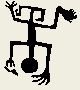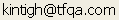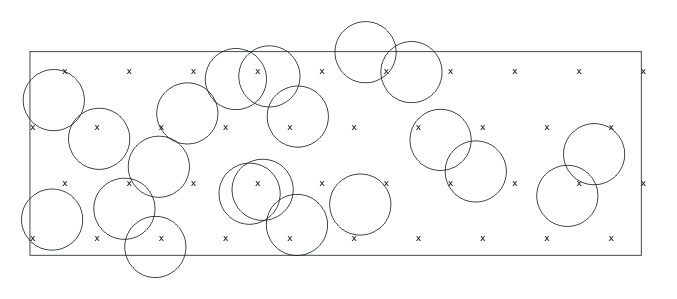Tools for Quantitative Archaeology    Email:STP: Monte Carlo Evaluation of a Subsurface Testing Program

STP performs a Monte Carlo evaluation of an arbitrary layout of test units within a rectangular survey area. The program empirically determines the probability that the layout of test units would detect a circular site with any given diameter, any artifact density, and any of several different artifact density distributions across the site. If the computer has a display compatible with the IBM Color Graphics Adapter, the program will graphically display its operation (at a considerable cost in speed). The conceptual basis of the program and preliminary results are presented in Kintigh (1986) and are not repeated here. This section presents detailed information on the operation of the program.

While STP is quite flexible, it has a number of limits. The principal limit of consequence is that the survey area can contain a maximum of 1000 test units. However, this limit can be circumvented by breaking up a single survey area into several pieces and evaluating them separately. Also, large numbers of test units in the survey area are to be avoided because of the large computation time required.

OPERATION OF STP

The STP program is started from DOS. The program then prompts the user for all information that it needs to run, including the name of the file in which the survey area description is contained. The prompts issued by the program are described below. The program provides sensible default responses to many prompts. For more information about the conventions used in these prompts, see the section entitled "Program Conventions."

To start STP type: STP<Enter>.

Specify Random Number Seed Value {N} ?

The first prompt asks you if you want to set the random number generator seed. In almost all cases the default reply of N (or <Enter>) will be desired. In general, the random number generator will produce a different sequence of random numbers for each run, which is what you would usually like. However, if you want to reproduce a previous run exactly, reply Y to this prompt, provide the random number seeds (in the same order) from the previous run and answer the remaining prompts in the same way as you did in the previous run.

If you reply N (or <Enter>) the program will tell you the random number seeds it is using:

Random Seed: 61925134

Display Graphics (IBM Color Card Only) {N} ?

The program now asks if you want to display the operation of the program graphically. If you have a graphics adapter compatible with the IBM CGA, EGA, or VGA you can use this option. If you can use it, this option is useful both for making sure that the survey area and shovel test pit arrangements look right, and for observing the operation of the program. However, using this option the program will run only at about 1/4 the speed of that it would without the graphic display and without a math co-processor. Once you are familiar with the program and have examined you data, you won't want to use this option.

If you use the graphic display, you obtain a more limited set of prompts than you would without it. The full set of prompts is listed below.

[S]ingle or [B]atched Runs {S} ?

This prompt is issued only when you have requested the graphic mode and in most cases the default reply of S is desired. This prompt asks whether you want to specify a number of different evaluations (runs) in advance, i.e. you want to Batch the runs, or whether you want to specify them Singly as you go along.

Output File for Results {.LST} ?

If you are not in graphic mode, the program places the results of its calculations in a disk file. Reply to this prompt with the name of the file in which the results should be placed.

Number of Repeated Simulation Trials {1} ?

In most cases, the default reply of 1 (also obtained by <Enter>) will be desired; read on only if you want all of the gory details. This prompt is not requesting the number of Monte Carlo trials that you want to run; it is asking how many times you want to run each specified evaluation. Replies other than the default can be used for obtaining results on the distribution of testing program results (see the section, Distribution of Testing Program Results, in Kintigh 1986). For example, if you wanted to know the probability that 1 site of a given description would be detected when 5 sites are actually present, you might do 200 trials (i.e. reply 200 to this prompt) each with 5 hypothetical (randomly located) sites (see below). However, note that the program only lists the result of each trial and does not tabulate the desired number directly; to accomplish the desired task, you would probably want to edit the program listing and read the program output into a statistical package to perform the desired analysis. Note also that, in general, the distribution will follow a binomial distribution which can be calculated directly.

Must Hypothetical Sites Have Centers within the Survey Area {Y} ?

In nearly all cases, the default reply of Y (also obtained by <Enter>), will be desired. Sorry, this is another obscure option. See the discussion in Kintigh (1986) under section, The Computer Program.

Number of Hypothetical Sites in Each Trial {1000} ?

This prompt requests the number of randomly located sites that are simulated for each evaluation. The probability of intersecting and detecting a site is estimated by the proportion of simulated sites that is intersected or detected. The larger the number of hypothetical (simulated) sites, the greater the accuracy of these probability estimates. Thus, a relatively small number of hypothetical sites (e.g. 200) may be sufficient to get a rough idea of the site detection probabilities, a much larger number of simulations (e.g. 10,000) may be needed to resolve small differences in detection probabilities between two different testing strategies.

Number of Different Test Unit Layouts to Try {1} ?

Survey Area Boundary & Test Unit Location File {.DAT} ?

Because the program can take quite a long time to do its evaluations, it allows you to specify a large number of different evaluations for it to perform without further intervention from you. If you want to evaluate a single test unit layout, simply reply 1 or <Enter> to the first prompt. However, if you want to compare two different layouts of test units (e.g. a hexagonal and a grid layout), under the same sets of assumptions you should answer 2 here.

For the number of layouts specified in the first prompt, in the second prompt the program asks you for the name of the file that describes the survey area and layout. Each file must first list the x and y coordinates of the four corners of a rectangular survey area, starting with the southwest corner, and proceeding counterclockwise. These 8 numbers (4 x-y pairs) can appear on any number of lines in any reasonable format. After this, the program reads sets of three numbers that describe each test unit. These three numbers are the x and y coordinates of the test unit and the test unit area (in the same unit of measure as the coordinates). A sample input file is listed in a subsequent section.

As each file name is entered, the program will read through the file and report what it finds, e.g.:

4 Corners & 23 Test Unit Locations Read

Number of Site Sizes to Try {1} ?

Site Diameter ?

The first prompt requests the number of different site sizes you wish to evaluate. Then it asks for that number of site diameters. A separate Monte Carlo evaluation is performed for each layout for each of the site sizes specified here.

Number of Densities to Try {1} ?

Average Artifact Density ?

As with the site sizes, the program asks how many different artifact densities you want to evaluate for each site size for each layout. It then asks for that number of densities expressed as a count per unit area (e.g., if the coordinates are in meters, the densities are in square meters).

If not all artifacts excavated would be noticed, instead of the actual density enter the effective density. The effective density is the actual density times the probability of discovering an artifact actually present. Thus if 80% of all artifacts in a sample are discovered and the actual density is 10, the effective density is 8.

Number of Density Function Shapes {1} ?

Shape: [U]niform [H]emisphere [C]onic [S]ine [N]eg. Binomial ?

Negative Binomial k {1.0} ?

As with the site sizes and artifact densities, the program, asks how many density function shapes you wish to evaluate for each layout, site size, and artifact density. Then for the number of density function shapes that you list, the program asks for the density function letter code. If you ask for a negative binomial density function, the program asks for the negative binomial parameter k. With the negative binomial distribution function, each different value of k specified is considered a different shape. Thus if you wish to consider uniform, negative binomial k=.5, and negative binomial k=2, you should reply 3 to the number of density function shapes.

Unlike the other distribution functions, the negative binomial distribution function does not have a direct geometric interpretation. Basically, the negative binomial function with a positive parameter will simulates a patchy distribution. The smaller the k, the greater the patchiness (and the harder the site is to detect). Based on real-world studies, it is probably reasonable to use k values between 0.2 to 5 (see Nance 1983; McManamon 1984). K cannot be 0, and negative k values simulate a uniform distribution.

Now the program lists information relevant to the amount of computation required and gives a rough time estimate. E.g.,

Number of Sites Placements Simulated: 2000

Number of Site-Test Unit Comparisons: 46000

Estimated time: 3 Minutes

OK to Proceed {Y} ?

Finally the program asks if it is OK to proceed. If so, just hit <Enter> or Y. If you have made a mistake or if the time estimate is too long for you can reenter the necessary values. As the program proceeds with its computation, it gives you some idea of how it is progressing, in terms of the percentage of all computation that has been completed and the total time elapsed. However, this display is updated only after each evaluation, and so it may appear that nothing is happening for relatively lengthy periods. Have patience.

100% 1.51 Minutes Elapsed

Execution Time 1.51 Minutes

SAMPLE SESSION

```Specify Random Number Seed Value {N} ?
Random Seed: 61925134
Display Graphics (IBM Color Card Only) {N} ?
Output File for Results {.LST} ? test
Number of Repeated Simulation Trials {1} ?
Must Hypothetical Sites Have Centers within the Survey Area {Y} ?
Number of Hypothetical Sites in Each Trial {1000} ? 500
Number of Different Test Unit Layouts to Try {1} ?
Survey Area Boundary & Test Unit Location File {.DAT} ? test
4 Corners & 23 Test Unit Locations Read
Number of Site Sizes to Try {1} ? 2
Site Diameter ? 10
Site Diameter ? 20
Number of Densities to Try {1} ? 2
Average Artifact Density ? 1
Average Artifact Density ? 10
Number of Density Function Shapes {1} ? 1
Shape: [U]niform [H]emisphere [C]onic [S]ine [N]eg. Binomial ? S
Number of Sites Placements Simulated:      2000
Number of Site-Test Unit Comparisons:     46000
Estimated time: 3 Minutes
OK to Proceed {Y} ?

100%     1.51 Minutes Elapsed
Execution Time   1.51 Minutes
```

SAMPLE OUTPUT

Reproduced below is the file TEST.DAT produced by the interactive session listed above using the data displayed as the sample output from PLACESTP. The heading lists information about the run so that once you mix up your printouts you can still see what your hours of computation gained you.

Each row represents a Monte Carlo evaluation of a test unit layout for a site size, artifact density, and artifact density distribution. The columns give the information that defines each separate evaluation and gives its results. First the sequential number of the file containing the layout that is being evaluated (this is printed just above, but if the headings are edited out for further analysis, this identifier is helpful). The second column lists the site diameter. The next three columns list the characteristics of the artifact scatter, its distribution function, S=sinusoidal, etc., and its average and maximum densities. The number of Monte Carlo trials (hypothetical sites simulated for each evaluation) is in the column headed No. Sites.

The results are given in the following 6 columns. The first three list the number of sites intersected by at least on test unit, the percentage of sites intersected by at least one test unit, and the number of intersection "hits", test units that intersect sites. For sites smaller than the interval between the test units, the count of sites intersected by test units and test units intersecting sites will be the same. However, if sites are larger than the test unit interval, then a site may be intersected by more than one test unit and the count may be higher than the number of sites. Similarly, the number and percentage of sites actually detected by the test unit layout (that is taking the artifacts into account) are given in the next two columns, and the detection hits (the number of test units that detect sites) is given in the final column.

```STP: Kintigh's Subsurface Testing Evaluation
Output File:TEST.LST    Random Seeds: 6192, 5134

3/26/1987 - 20:15:20
Input File: TEST.DAT

File  Site   Artifact Density     No.   Sites Intersected      Sites Detected
No.  Diam  Fn Average Maximum  Sites  Number   Pct   Hits  Number   Pct   Hits
1    10   S     1.0     3.4    500      84  16.8     84      11   2.2     11
1    10   S    10.0    33.6    500      87  17.4     87      41   8.2     41
1    20   S     1.0     3.4    500     327  65.4    327      42   8.4     42
1    20   S    10.0    33.6    500     309  61.8    309     156  31.2    156
Execution Time   1.5 Minutes```Graphic Display of STP Program. On the screen, the circles will appear in different colors or with different line types depending upon whether or not they "located" any artifacts.

GRAPHIC DISPLAY

The graphic display (see example) of the program results is described briefly, below. The survey area boundary is shown by the rectangle, and the test units are shown as crosses within the survey area. Hypothetical sites are the circles shown; heavy circles are sites that are not detected, lighter circles are those that are detected. The diameter of the hypothetical sites is given at the end of the first text line. The number of hypothetical sites is counted off at the left of the second line, while the density distribution letter code and artifact density are given to the right. The third and fourth textual lines give the number and percentage of sites intersected and detected and the count of test units that intersect or detect sites (see sample output, above). Pressing any key will stop the simulation process. Note if after the program terminates you get ugly characters on your screen, put in the DOS disk and type MODE MONO.

Below the graphic display on the screen, the program progress is displayed as follows:

Test Unit Evaluation: Site Dia 15.0

Site 20 U Density 10.0

Intersect 4 20% Counted 4

Detect 1 5% Counted 1

NOTES ON PROGRAM OPERATION

The main factor that determines time required for the program to run is the number of site-test unit comparisons. Evaluation of a given test unit layout for a fixed site size, artifact density, and artifact density distribution requires that each hypothetical site simulated be compared with each test unit to see if the test unit intersects the site. Thus, if there are 100 test units and 1000 hypothetical sites generated for each evaluation, the evaluation requires 100,000 site-test unit comparisons.

Separate evaluations are done for each combination of layout, site size, artifact density, and artifact density distribution. Thus if you request evaluation of 3 layouts for 5 site sizes, 5 densities, and 4 density distributions, you have requested 3*5*5*4=300 separate evaluations, each of which requires 100,000 comparisons, for a total of 30,000,000 comparisons.

Experimentation suggests that if a systematic testing strategy is being used on a large area, only a portion of that area can be examined with little effect on the results. For example with a 10 km right- of-way 120 m wide, it is probably sufficient to look at a 500 m section of the right of way.

 Home Top Overview Ordering Documentation

Page Last Updated - 22-Jul-2007